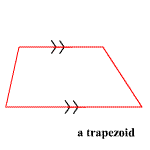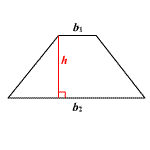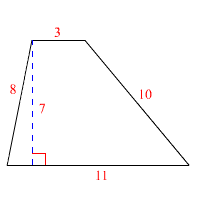# Trapezoids: Area and Perimeter

A trapezoid , also called a trapezium in some countries, is a quadrilateral with exactly one pair of parallel sides.The parallel sides are called the bases and the non-parallel sides are the legs of the trapezoid.

An isosceles trapezoid is a trapezoid in which the two non-parallel sides are congruent .

The area $A$ of a trapezoid is given by

$A=\frac{{b}_{1}+{b}_{2}}{2}h$

where ${b}_{1}$ and ${b}_{2}$ are the lengths of the two parallel sides, and $h$ is the height, as shown in the figure below.The perimeter of a trapezoid is the sum of the lengths of its four sides. If one or more of the lengths is not known, you can sometimes use the Pythagorean Theorem to find it.

Example:

Find the area and perimeter of the trapezoid shown.To find the area, apply the formula.

$\begin{array}{l}A=\frac{{b}_{1}+{b}_{2}}{2}h\\ =\frac{3+11}{2}\left(7\right)\\ =7\left(7\right)\\ =49\text{\hspace{0.17em}}\text{\hspace{0.17em}}\text{square}\text{\hspace{0.17em}}\text{\hspace{0.17em}}\text{units}\end{array}$

To find the perimeter, add the lengths of all four sides.

$\begin{array}{l}P=3+10+11+8\\ =32\text{\hspace{0.17em}}\text{\hspace{0.17em}}\text{units}\end{array}$